Short articles on topics in systematic trading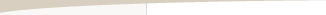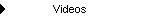Other Articles...

Fixed ratio position sizingFixed Ratio Position Sizing

by Michael R. Bryant

In fixed ratio position sizing the key parameter is the delta. This is the dollar amount of profit per contract to increase the number of contracts by one. A delta of \$3,000, for example, means that if you're currently trading one contract, you need to increase your account equity by \$3,000 to start trading two contracts. Once you get to two contracts, you need an additional profit of \$6,000 to start trading three contracts. At three contracts, you would need an additional profit of \$9,000 to start trading four contracts, and so on. For stock trading, a "contract" can be interpreted as a fixed number of shares, such as 100 shares.

Fixed ratio position sizing was developed by Ryan Jones in his book "The Trading Game," John Wiley & Sons, New York, 1999. Based on an equation presented by Jones, it's possible to derive the following equation for the number of contracts in fixed ratio position sizing:

N = 0.5 * [(1 + 8 * P/delta)^0.5 + 1]

where N is the number of contracts, P is the total closed trade profit, and delta is the parameter discussed above. The carat symbol (^) represents exponentiation; that is, the quantity in parentheses is raised to the power of 0.5 (square root).

A few points are worth noting. The profit, P, is the accumulated profit over all trades leading up to the one for which you want to calculate the number of contracts. Consequently, the number of contracts for the first trade is always one because you always start with zero profits (P = 0). Also, as you accrue more profits, the number of contracts increases more slowly. A \$10,000 profit made early in a sequence of trades will increase the number of contracts more than a \$10,000 profit made after many other profitable trades.

Unlike with fixed fractional trading, the trade risk is not a factor in the fixed ratio equation. All that matters is the accumulated profit and the delta. The delta determines how quickly the contracts are added or subtracted. Also note that the account equity is not a factor. Changing the starting account size, for example, will not change the number of contracts, provided there is enough equity to continue trading.

As an example, consider the series of trades below. The starting account size was \$50,000. The profit/loss per contract is shown in the second column ("PL/Contr"). The next column is the trade risk, which is not used in fixed ratio position sizing. The delta was \$1500. The next column shows the number of contracts computed according the equation above. Multiplying the number of contracts by the profit/loss per contract results in the position profit/loss ("Pos PL"), which adds to the current equity value to give the new value of account equity, shown in the last column.Trades and number of contracts in an example of fixed ratio position sizing.

Notice how the number of contracts starts at one and slowly increases as profits accumulate. If a smaller delta had been used, the number of contracts would have increased more quickly but would have dropped more after a loss.

The equity curve and the number of contracts is shown below over a longer span of trades from the same market system. The bar chart, below the equity curve, illustrates how the number of contracts increases with increasing profits.Equity curve and number of contracts in an example of fixed ratio position sizing.

Because the number of contracts always starts at one, the fixed ratio method usually produces better results for smaller accounts. For larger accounts, it may take an unreasonable amount of time to increase the number of contracts to a level that takes full advantage of the available equity.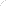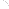Join Our Email List Email: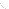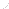For Email Marketing you can trust